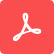# Sources

By Emerson Melo, Tom Chen and Xin Lei

This tutorial covers the sources of electromagnetic fields in FDTD simulations. You will learn about the various types of sources commonly used to drive specific simulations and how to set them up to build your FDTD models.

Share On:
Additional information: This Tutorial was updated in Aug 16, 2023Tidy3D GUI: Tutorial 4

Hello! It's time to discuss the sources of electromagnetic fields in FDTD simulations. In this tutorial, you'll learn about the different types of sources commonly used and how to set them up to build your FDTD models.

So, once you've created an empty simulation, add a new source and name it "dipole". Then, from the "type" drop-down list, select the "PointDipole" option.

This will allow you to simulate oscillating dipoles, which are useful for exciting resonant modes of cavities or calculating photonic crystal band structures. They can also model light emission from fluorescent molecules. You can leave the dipole at its default position, or change it as desired.

Next, define the source field pattern by selecting one of the electric or magnetic field components.

These animations demonstrate how the direction of the injected electromagnetic waves changes when you select different point dipole polarizations.

In addition to the field pattern, you need to set the time-dependency of the source. You can achieve this by choosing a wavelength range, as shown here, or a frequency center and bandwidth.

Click the "Plot" button to visualize the source spectrum and its time-dependence. You can further adjust source bandwidth using the “Customize source bandwidth” parameter, but usually that is not necessary.

If necessary, you can adjust the source amplitude, phase, and the position of its maximum value in the time domain using the "Advanced" tab.

Finally, create a field monitor and run the simulation to observe the field pattern created by the point dipole.

Moving forward, use the "Edit" command to create a new simulation version. Then, disable the point dipole source and add a new source. Choose the "UniformCurrentSource" type, which is similar to the point dipole source but generalized to higher dimensions.

So, let's create a line source, as shown in this animation. Set its size to 9 microns in the x-direction, select the "Ex" polarization, and adjust the time dependence as before. Run the simulation and visualize the results.

For certain problems like calculating the response of metasurfaces and diffraction gratings, it's necessary to have uniform illumination at different incident angles. In such cases, you can add a "PlaneWave" source, as demonstrated here.

Here, the "direction" parameter sets a downward or upward wave, the "angle_theta" and "angle_phi" represent the polar and azimuth angles of the propagation direction, respectively. You can switch between S and P polarizations using the "polar_angle" parameter.

When computing scattered fields only, such as when calculating scattered cross sections of various objects, you can add a total-field scattered-field (TFSF) source. As shown in these animations, the TFSF source allows you to specify a box region where a plane wave is injected. Inside this region, the fields are a superposition of the incident and scattered waves, while outside the TFSF box, you have only the scattered fields.

The setup for TFSF is similar to the plane wave one, but you need to define a volume region and set the field injection axis.

A GaussianBeam source is the ideal choice for simulating the coupling between electromagnetic devices and optical fibers or lenses.

The new parameters here are the "waist_radius", which determines the spot size of the beam at the focal point, and the "waist_distance", which must be negative for a converging beam and positive for a diverging one. An "AstigmaticGaussianBeam" source is also available for cases where the waist radius should be different in the x- and y-directions.

Concluding, to excite a device using a specific guided mode, like shown here, you need to use a "ModeSource". For a simple example, create a waveguide that is 1.5 microns wide and 0.75 microns thick, with a refractive index of 2.0. Include a mode source in the simulation and set its position and size as shown here. Let's insert the TM1 guided mode, where the electric field oscillates in the z-direction.

So, set the "mode_index" parameter to 3 to select it. In this case, a "num_modes" value of 4 is necessary, as the modes are sorted by their effective indices in descending order. The "target_neff" parameter is a guess for the desired mode effective index. In this example, you can set it to the waveguide refractive index to start from the highest effective index mode, which is the TE0.

After running the simulation, you'll observe the TM1 mode propagating through the waveguide in the positive x-direction. Now, you know how to select and set up a light source for different situations. In the next tutorials, you'll learn how to set up monitors to calculate and visualize quantities of interest in FDTD simulations.